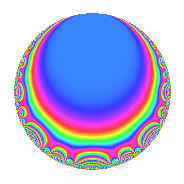# Properties

 Label 8008.2.a.iLevel 8008 Weight 2 Character orbit 8008.a Self dual Yes Analytic conductor 63.944 Analytic rank 1 Dimension 3 CM No Inner twists 1

# Related objects

## Newspace parameters

 Level: $$N$$ = $$8008 = 2^{3} \cdot 7 \cdot 11 \cdot 13$$ Weight: $$k$$ = $$2$$ Character orbit: $$[\chi]$$ = 8008.a (trivial)

## Newform invariants

 Self dual: Yes Analytic conductor: $$63.9442019386$$ Analytic rank: $$1$$ Dimension: $$3$$ Coefficient field: 3.3.229.1 Coefficient ring: $$\Z[a_1, a_2, a_3]$$ Coefficient ring index: $$1$$ Fricke sign: $$1$$ Sato-Tate group: $\mathrm{SU}(2)$

## $q$-expansion

Coefficients of the $$q$$-expansion are expressed in terms of a basis $$1,\beta_1,\beta_2$$ for the coefficient ring described below. We also show the integral $$q$$-expansion of the trace form.

 $$f(q)$$ $$=$$ $$q$$ $$+ ( 1 + \beta_{2} ) q^{3}$$ $$+ ( 2 - \beta_{1} ) q^{5}$$ $$- q^{7}$$ $$+ ( 1 + \beta_{1} ) q^{9}$$ $$+O(q^{10})$$ $$q$$ $$+ ( 1 + \beta_{2} ) q^{3}$$ $$+ ( 2 - \beta_{1} ) q^{5}$$ $$- q^{7}$$ $$+ ( 1 + \beta_{1} ) q^{9}$$ $$- q^{11}$$ $$+ q^{13}$$ $$+ ( 1 - 2 \beta_{1} + 2 \beta_{2} ) q^{15}$$ $$+ ( -5 + \beta_{1} - 2 \beta_{2} ) q^{17}$$ $$+ ( 2 \beta_{1} - \beta_{2} ) q^{19}$$ $$+ ( -1 - \beta_{2} ) q^{21}$$ $$+ ( -4 + 3 \beta_{1} + \beta_{2} ) q^{23}$$ $$+ ( 2 - 4 \beta_{1} + \beta_{2} ) q^{25}$$ $$+ ( -1 + 2 \beta_{1} - 2 \beta_{2} ) q^{27}$$ $$+ ( 2 - 2 \beta_{1} - 2 \beta_{2} ) q^{29}$$ $$+ ( -6 + \beta_{1} - \beta_{2} ) q^{31}$$ $$+ ( -1 - \beta_{2} ) q^{33}$$ $$+ ( -2 + \beta_{1} ) q^{35}$$ $$+ ( 2 + \beta_{1} - \beta_{2} ) q^{37}$$ $$+ ( 1 + \beta_{2} ) q^{39}$$ $$+ ( -2 - 6 \beta_{1} + 4 \beta_{2} ) q^{41}$$ $$+ ( 3 + 3 \beta_{1} ) q^{43}$$ $$+ ( -1 + \beta_{1} - \beta_{2} ) q^{45}$$ $$+ ( -4 \beta_{1} + 2 \beta_{2} ) q^{47}$$ $$+ q^{49}$$ $$+ ( -10 - 3 \beta_{2} ) q^{51}$$ $$+ ( -5 - \beta_{1} - 4 \beta_{2} ) q^{53}$$ $$+ ( -2 + \beta_{1} ) q^{55}$$ $$+ ( -1 + 3 \beta_{1} + \beta_{2} ) q^{57}$$ $$+ ( 2 + 3 \beta_{1} - 3 \beta_{2} ) q^{59}$$ $$+ ( 3 + \beta_{1} ) q^{61}$$ $$+ ( -1 - \beta_{1} ) q^{63}$$ $$+ ( 2 - \beta_{1} ) q^{65}$$ $$+ ( -3 - 2 \beta_{1} + \beta_{2} ) q^{67}$$ $$+ ( 2 + 7 \beta_{1} - 5 \beta_{2} ) q^{69}$$ $$+ ( -8 + \beta_{1} - 2 \beta_{2} ) q^{71}$$ $$+ ( -6 - 4 \beta_{1} ) q^{73}$$ $$+ ( 1 - 7 \beta_{1} + \beta_{2} ) q^{75}$$ $$+ q^{77}$$ $$+ ( 2 \beta_{1} + 3 \beta_{2} ) q^{79}$$ $$+ ( -8 - \beta_{1} + \beta_{2} ) q^{81}$$ $$+ ( 4 \beta_{1} + \beta_{2} ) q^{83}$$ $$+ ( -11 + 9 \beta_{1} - 5 \beta_{2} ) q^{85}$$ $$+ ( -6 - 6 \beta_{1} + 4 \beta_{2} ) q^{87}$$ $$+ ( 5 + 4 \beta_{1} - \beta_{2} ) q^{89}$$ $$- q^{91}$$ $$+ ( -8 + \beta_{1} - 5 \beta_{2} ) q^{93}$$ $$+ ( -5 + 5 \beta_{1} - 4 \beta_{2} ) q^{95}$$ $$+ ( -2 + 5 \beta_{1} - 7 \beta_{2} ) q^{97}$$ $$+ ( -1 - \beta_{1} ) q^{99}$$ $$+O(q^{100})$$ $$\operatorname{Tr}(f)(q)$$ $$=$$ $$3q$$ $$\mathstrut +\mathstrut 2q^{3}$$ $$\mathstrut +\mathstrut 6q^{5}$$ $$\mathstrut -\mathstrut 3q^{7}$$ $$\mathstrut +\mathstrut 3q^{9}$$ $$\mathstrut +\mathstrut O(q^{10})$$ $$3q$$ $$\mathstrut +\mathstrut 2q^{3}$$ $$\mathstrut +\mathstrut 6q^{5}$$ $$\mathstrut -\mathstrut 3q^{7}$$ $$\mathstrut +\mathstrut 3q^{9}$$ $$\mathstrut -\mathstrut 3q^{11}$$ $$\mathstrut +\mathstrut 3q^{13}$$ $$\mathstrut +\mathstrut q^{15}$$ $$\mathstrut -\mathstrut 13q^{17}$$ $$\mathstrut +\mathstrut q^{19}$$ $$\mathstrut -\mathstrut 2q^{21}$$ $$\mathstrut -\mathstrut 13q^{23}$$ $$\mathstrut +\mathstrut 5q^{25}$$ $$\mathstrut -\mathstrut q^{27}$$ $$\mathstrut +\mathstrut 8q^{29}$$ $$\mathstrut -\mathstrut 17q^{31}$$ $$\mathstrut -\mathstrut 2q^{33}$$ $$\mathstrut -\mathstrut 6q^{35}$$ $$\mathstrut +\mathstrut 7q^{37}$$ $$\mathstrut +\mathstrut 2q^{39}$$ $$\mathstrut -\mathstrut 10q^{41}$$ $$\mathstrut +\mathstrut 9q^{43}$$ $$\mathstrut -\mathstrut 2q^{45}$$ $$\mathstrut -\mathstrut 2q^{47}$$ $$\mathstrut +\mathstrut 3q^{49}$$ $$\mathstrut -\mathstrut 27q^{51}$$ $$\mathstrut -\mathstrut 11q^{53}$$ $$\mathstrut -\mathstrut 6q^{55}$$ $$\mathstrut -\mathstrut 4q^{57}$$ $$\mathstrut +\mathstrut 9q^{59}$$ $$\mathstrut +\mathstrut 9q^{61}$$ $$\mathstrut -\mathstrut 3q^{63}$$ $$\mathstrut +\mathstrut 6q^{65}$$ $$\mathstrut -\mathstrut 10q^{67}$$ $$\mathstrut +\mathstrut 11q^{69}$$ $$\mathstrut -\mathstrut 22q^{71}$$ $$\mathstrut -\mathstrut 18q^{73}$$ $$\mathstrut +\mathstrut 2q^{75}$$ $$\mathstrut +\mathstrut 3q^{77}$$ $$\mathstrut -\mathstrut 3q^{79}$$ $$\mathstrut -\mathstrut 25q^{81}$$ $$\mathstrut -\mathstrut q^{83}$$ $$\mathstrut -\mathstrut 28q^{85}$$ $$\mathstrut -\mathstrut 22q^{87}$$ $$\mathstrut +\mathstrut 16q^{89}$$ $$\mathstrut -\mathstrut 3q^{91}$$ $$\mathstrut -\mathstrut 19q^{93}$$ $$\mathstrut -\mathstrut 11q^{95}$$ $$\mathstrut +\mathstrut q^{97}$$ $$\mathstrut -\mathstrut 3q^{99}$$ $$\mathstrut +\mathstrut O(q^{100})$$

Basis of coefficient ring in terms of a root $$\nu$$ of $$x^{3}\mathstrut -\mathstrut$$ $$4$$ $$x\mathstrut -\mathstrut$$ $$1$$:

 $$\beta_{0}$$ $$=$$ $$1$$ $$\beta_{1}$$ $$=$$ $$\nu$$ $$\beta_{2}$$ $$=$$ $$\nu^{2} - 3$$
 $$1$$ $$=$$ $$\beta_0$$ $$\nu$$ $$=$$ $$\beta_{1}$$ $$\nu^{2}$$ $$=$$ $$\beta_{2}\mathstrut +\mathstrut$$ $$3$$

## Embeddings

For each embedding $$\iota_m$$ of the coefficient field, the values $$\iota_m(a_n)$$ are shown below.

For more information on an embedded modular form you can click on its label.

Label $$\iota_m(\nu)$$ $$a_{2}$$ $$a_{3}$$ $$a_{4}$$ $$a_{5}$$ $$a_{6}$$ $$a_{7}$$ $$a_{8}$$ $$a_{9}$$ $$a_{10}$$
1.1
 −0.254102 −1.86081 2.11491
0 −1.93543 0 2.25410 0 −1.00000 0 0.745898 0
1.2 0 1.46260 0 3.86081 0 −1.00000 0 −0.860806 0
1.3 0 2.47283 0 −0.114908 0 −1.00000 0 3.11491 0
 $$n$$: e.g. 2-40 or 990-1000 Significant digits: Format: Complex embeddings Normalized embeddings Satake parameters Satake angles

## Inner twists

This newform does not admit any (nontrivial) inner twists.

## Atkin-Lehner signs

$$p$$ Sign
$$2$$ $$-1$$
$$7$$ $$1$$
$$11$$ $$1$$
$$13$$ $$-1$$

## Hecke kernels

This newform can be constructed as the intersection of the kernels of the following linear operators acting on $$S_{2}^{\mathrm{new}}(\Gamma_0(8008))$$:

 $$T_{3}^{3}$$ $$\mathstrut -\mathstrut 2 T_{3}^{2}$$ $$\mathstrut -\mathstrut 4 T_{3}$$ $$\mathstrut +\mathstrut 7$$ $$T_{5}^{3}$$ $$\mathstrut -\mathstrut 6 T_{5}^{2}$$ $$\mathstrut +\mathstrut 8 T_{5}$$ $$\mathstrut +\mathstrut 1$$ $$T_{17}^{3}$$ $$\mathstrut +\mathstrut 13 T_{17}^{2}$$ $$\mathstrut +\mathstrut 37 T_{17}$$ $$\mathstrut -\mathstrut 28$$